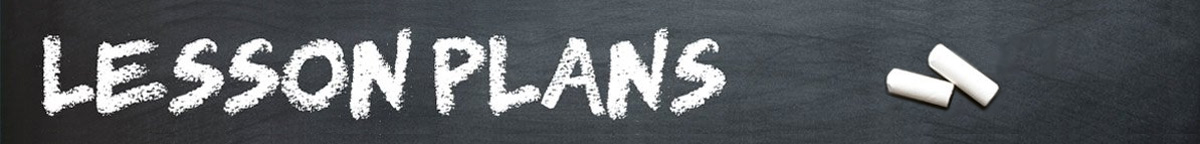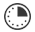# Order in the Math Class

JRDAssist
Middle School and High School Teacher

The students will physically review the order of operations.

##Length of Time: 1-2 Class Periods

Featured Programs:

### Common Core Alignment

CCSS.Math.Content.6.EE.A.2.C - Evaluate expressions at specific values of their variables. Include expressions that arise from formulas used in real-world problems. Perform arithmetic operations, including those involving whole-number exponents, in the conventional order when there are no parentheses to specify a particular order (Order of Operations). For example, use the formulas V = s3 and A = 6 s2 to find the volume and surface area of a cube with sides of length s = 1/2.

## Objectives & Outcomes

Using an interactive process, the students will be able to correctly use the order of operations.

## Materials Needed

Pre-cut sheets of paper/cardboard large enough to see at a short distance, markers

Prepare ahead of time:  Create about 15 pieces of cardboard/paper with each having a different symbol, number, number w/exponent, root, parenthesis, etc.

## Procedure

### Opening to Lesson

• Display the letters: PEMDAS (Parenthesis, Exponents, Multiplication, Addition, Subtraction)
• Explain to students this will help them remember the order of operations
• Display an example and have students solve the problem
• Discuss responses and common errors

### Body of Lesson

#### Modeling

• Display prepared pieces of paper/cardboard showing different parts of an expression: operation signs, exponents, parenthesis, etc.
• Give the samples to student volunteers or assign each to a student
• Have students stand in front of the room creating an acceptable expression to be solved
• Ask seated students to solve the problem
• Discuss responses and common errors
• Have the students shuffle the display
• Ask students to solve the new problem

#### Guided Practice

• Split students into two large groups
• Distribute blank sheets or paper/cardboard and markers to the students
• Have students create various operational signs, numbers, parenthesis, exponents, roots, etc. (This should take no more than 10-15 minutes)
• Once both groups have completed creating the display cards, tell students they will arrange them in a logical order for the other half of the class to solve
• Some students may be holding a parenthesis and a number, or an operational sign and number
• Encourage students to display easier problems first, then increase the difficulty
• Allow about 10-15 minutes for each group to present the problems in front of the classroom
• The seated students will be solving the expression (An option may be for the class to solve together the first couple of times.)
• During the lesson, remind students of PEMDAS, but also tell students that when it is only addition/subtraction or multiplication/division, work left to right
• Seated students may also write out the problem to help with solving
• Review PEMDAS

#### Independent Practice

• Create or use a commercial worksheet with problems students will solve using the correct order of operations, in-class or for homework.

### Closing

Ask students why it is important to solve problems using the correct order of operations.  Finally, ask students to create a new pneumonic device to recall the order of operations.

## Assessment & Evaluation

Assessment page used for students to solve order of operation expressions/problems

## Modification & Differentiation

Students work in smaller groups; have the paper/cardboard symbols, numbers, etc. created ahead of time and distribute some to each student randomly calling students to the front of the room to display different problems; do the lesson outdoors or in a gym where there is more room; have students create a 6-foot problem using old adding machine-like tape for peers to solve.

## Related Lesson Plans

##### Interest-ing Math

Students will learn about simple interest and how to calculate the real cost of a loan, credit card, and other types of borrowing.

##### Prices and Percentages

Using a current list of prices for food and clothing, the students will practice math skills related to percentages.

##### Silent Geometry

The students will practice using geometry formulas measuring items in the classroom to find area and volume, radius and circumference, and identify the types of angles.

##### Ice Cream Cones, Baseballs, and Cans

Students will work in pairs to practice finding the volume of cones, cylinders, and spheres using everyday objects.